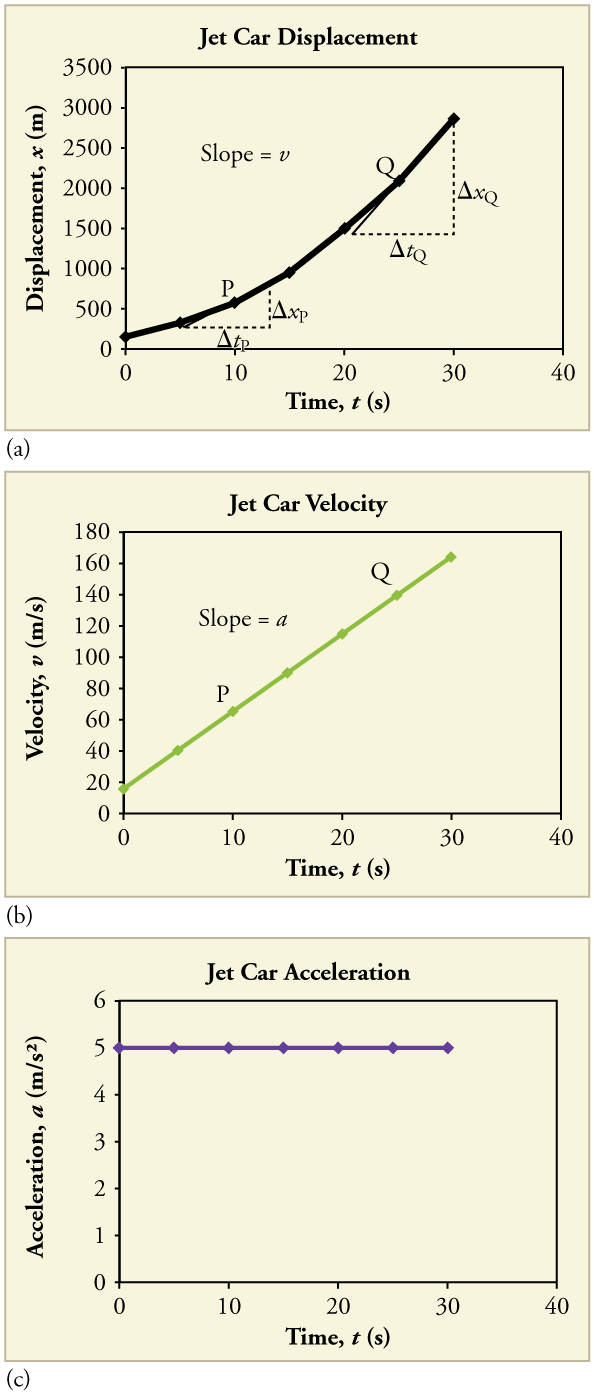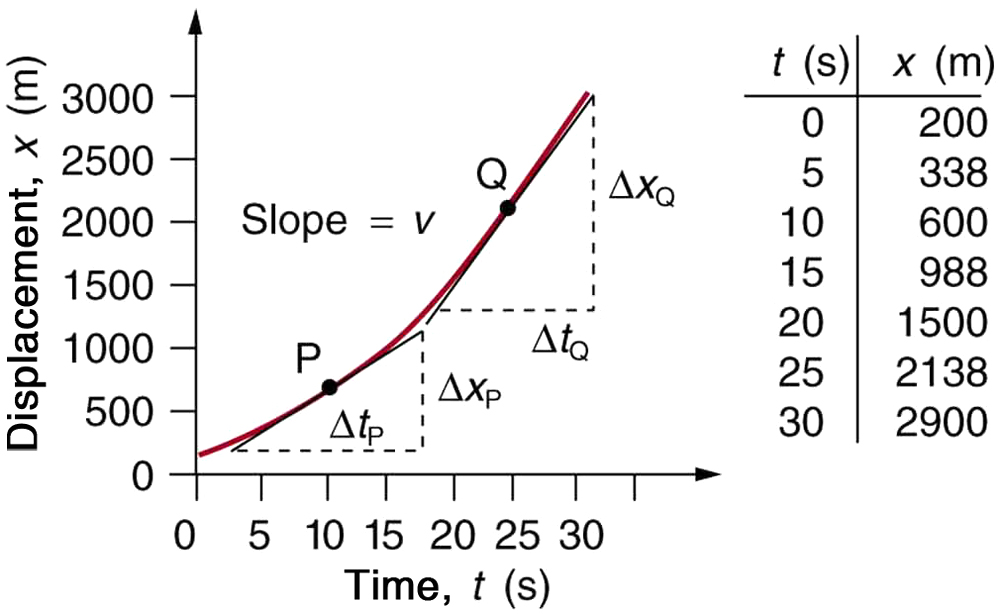# 2.8 Graphical analysis of one-dimensional motion  (Page 2/8)

 Page 2 / 8

## Graphs of motion when $a$ Is constant but $a\ne 0$

The graphs in [link] below represent the motion of the jet-powered car as it accelerates toward its top speed, but only during the time when its acceleration is constant. Time starts at zero for this motion (as if measured with a stopwatch), and the displacement and velocity are initially 200 m and 15 m/s, respectively.Graphs of motion of a jet-powered car during the time span when its acceleration is constant. (a) The slope of an x size 12{x} {} vs. t size 12{t} {} graph is velocity. This is shown at two points, and the instantaneous velocities obtained are plotted in the next graph. Instantaneous velocity at any point is the slope of the tangent at that point. (b) The slope of the v size 12{v} {} vs. t size 12{t} {} graph is constant for this part of the motion, indicating constant acceleration. (c) Acceleration has the constant value of 5 . 0 m/s 2 size 12{5 "." "0 m/s" rSup { size 8{2} } } {} over the time interval plotted.A U.S. Air Force jet car speeds down a track. (credit: Matt Trostle, Flickr)

The graph of displacement versus time in [link] (a) is a curve rather than a straight line. The slope of the curve becomes steeper as time progresses, showing that the velocity is increasing over time. The slope at any point on a displacement-versus-time graph is the instantaneous velocity at that point. It is found by drawing a straight line tangent to the curve at the point of interest and taking the slope of this straight line. Tangent lines are shown for two points in [link] (a). If this is done at every point on the curve and the values are plotted against time, then the graph of velocity versus time shown in [link] (b) is obtained. Furthermore, the slope of the graph of velocity versus time is acceleration, which is shown in [link] (c).

## Determining instantaneous velocity from the slope at a point: jet car

Calculate the velocity of the jet car at a time of 25 s by finding the slope of the $x$ vs. $t$ graph in the graph below.The slope of an x size 12{x} {} vs. t size 12{t} {} graph is velocity. This is shown at two points. Instantaneous velocity at any point is the slope of the tangent at that point.

Strategy

The slope of a curve at a point is equal to the slope of a straight line tangent to the curve at that point. This principle is illustrated in [link] , where Q is the point at $t=\text{25 s}$ .

Solution

1. Find the tangent line to the curve at $t=\text{25 s}$ .

2. Determine the endpoints of the tangent. These correspond to a position of 1300 m at time 19 s and a position of 3120 m at time 32 s.

3. Plug these endpoints into the equation to solve for the slope, $v$ .

$\text{slope}={v}_{Q}=\frac{{\Delta x}_{Q}}{{\Delta t}_{Q}}=\frac{\left(\text{3120 m}-\text{1300 m}\right)}{\left(\text{32 s}-\text{19 s}\right)}$

Thus,

${v}_{Q}=\frac{\text{1820 m}}{\text{13 s}}=\text{140 m/s.}$

Discussion

This is the value given in this figure’s table for $v$ at $t=\text{25 s}$ . The value of 140 m/s for ${v}_{Q}$ is plotted in [link] . The entire graph of $v$ vs. $t$ can be obtained in this fashion.

Carrying this one step further, we note that the slope of a velocity versus time graph is acceleration. Slope is rise divided by run; on a $v$ vs. $t$ graph, rise = change in velocity $\Delta v$ and run = change in time $\Delta t$ .

## The slope of v Vs. t

The slope of a graph of velocity $v$ vs. time $t$ is acceleration $a$ .

$\text{slope}=\frac{\Delta v}{\Delta t}=a$

Since the velocity versus time graph in [link] (b) is a straight line, its slope is the same everywhere, implying that acceleration is constant. Acceleration versus time is graphed in [link] (c).

how do you calculate the 5% uncertainty of 4cm?
4cm/100×5= 0.2cm
haider
how do you calculate the 5% absolute uncertainty of a 200g mass?
= 200g±(5%)10g
haider
use the 10g as the uncertainty?
melia
haider
topic of question?
haider
the relationship between the applied force and the deflection
melia
sorry wrong question i meant the 5% uncertainty of 4cm?
melia
its 0.2 cm or 2mm
haider
thank you
melia
Hello group...
Chioma
hi
haider
well hello there
sean
hi
Noks
the meaning of phrase in physics
is the meaning of phrase in physics
Chovwe
write an expression for a plane progressive wave moving from left to right along x axis and having amplitude 0.02m, frequency of 650Hz and speed if 680ms-¹
how does a model differ from a theory
To use the vocabulary of model theory and meta-logic, a theory is a set of sentences which can be derived from a formal model using some rule of inference (usually just modus ponens). So, for example, Number Theory is the set of sentences true about numbers. But the model is a structure together wit
Jesilda
with an iterpretation.
Jesilda
what is vector quantity
Vector quality have both direction and magnitude, such as Force, displacement, acceleration and etc.
Besmellah
Is the force attractive or repulsive between the hot and neutral lines hung from power poles? Why?
what's electromagnetic induction
electromagnetic induction is a process in which conductor is put in a particular position and magnetic field keeps varying.
Lukman
wow great
Salaudeen
what is mutual induction?
je
mutual induction can be define as the current flowing in one coil that induces a voltage in an adjacent coil.
Johnson
how to undergo polarization
show that a particle moving under the influence of an attractive force mu/y³ towards the axis x. show that if it be projected from the point (0,k) with the component velocities U and V parallel to the axis of x and y, it will not strike the axis of x unless u>v²k² and distance uk²/√u-vk as origin
show that a particle moving under the influence of an attractive force mu/y^3 towards the axis x. show that if it be projected from the point (0,k) with the component velocities U and V parallel to the axis of x and y, it will not strike the axis of x unless u>v^2k^2 and distance uk^2/√u-k as origin
No idea.... Are you even sure this question exist?
Mavis
I can't even understand the question
yes it was an assignment question "^"represent raise to power pls
Gabriel
Gabriel
An engineer builds two simple pendula. Both are suspended from small wires secured to the ceiling of a room. Each pendulum hovers 2 cm above the floor. Pendulum 1 has a bob with a mass of 10kg . Pendulum 2 has a bob with a mass of 100 kg . Describe how the motion of the pendula will differ if the bobs are both displaced by 12º .
no ideas
Augstine
if u at an angle of 12 degrees their period will be same so as their velocity, that means they both move simultaneously since both both hovers at same length meaning they have the same length
Modern cars are made of materials that make them collapsible upon collision. Explain using physics concept (Force and impulse), how these car designs help with the safety of passengers.
calculate the force due to surface tension required to support a column liquid in a capillary tube 5mm. If the capillary tube is dipped into a beaker of water
find the time required for a train Half a Kilometre long to cross a bridge almost kilometre long racing at 100km/h
method of polarization
Ajayi
What is atomic number?
The number of protons in the nucleus of an atom
Deborah

#### Get Jobilize Job Search Mobile App in your pocket Now!ByByBy OpenStaxBy OpenStaxBy Stephen VoronBy OpenStaxBy Donyea SweetsBy Anonymous UserBy OpenStaxBy OpenStaxBy OpenStaxBy